Learn from home

The teachers## Exercise 1

Calculate the head of the vector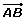knowing that its components are (3, −1) and its tail is A = (−2, 4).

## Exercise 2

Given points A = (0, a) and B = (1, 2), calculate the value of a if the magnitude of the vectoris one.

## Exercise 3

Normalize the vectors:= (1,),= (−4, 3) and= (8. −8).

## Exercise 4

Determine the unit vector,, which is in the same direction of the vector.

## Exercise 5

Calculate the coordinates of D so that the quadrilateral formed by the vertices: A = (−1, −2), B = (4, −1), C = (5, 2) and D; is a parallelogram.

## Exercise 6

The vectors= (1, 4) and= (1, 3) form a basis. Express this in basis the vector= (−1. −1).

## Exercise 7

Find the value of k so that the angle that forms between= (3, k) and= (2, −1) is:

1 90°

2

3 45°

## Exercise 8

Calculate the value of a so that the vectors= 3+ 4and= a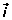− 2form an angle of 45°.

## Exercise 9

If {,} is an orthonormal basis, calculate:

1·2·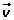3·4·## Solution of exercise 1

Calculate the head of the vectorknowing that its components are (3, −1) and its tail is A = (−2, 4).

3 = xB − (−2)xB = 1

−1 = yB − 4yB = 3

B(1, 3)

## Solution of exercise 2

Given points A = (0, a) and B = (1, 2), calculate the value of a if the magnitude of the vectoris one.## Solution of exercise 3

Normalize the vectors:= (1,),= (−4, 3) and= (8. −8).## Solution of exercise 4

Determine the unit vector,, which is in the same direction of the vector.## Solution of exercise 5

Calculate the coordinates of D so that the quadrilateral formed by the vertices: A = (−1, −2), B = (4, −1), C = (5, 2) and D; is a parallelogram.## Solution of exercise 6

The vectors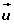= (1, 4) and= (1, 3) form a basis. Express in this basis the vector= (−1. −1).

(−1. −1) = a (1, 4) + b (1, 3)

−1 = a +b a = −1 −b a= 2

−1 = 4a +3b −1 = 4( −1 −b) +3b b = −3= 2− 3## Solution of exercise 7

Find the value of k so that the angle that forms between= (3, k) and= (2, −1) is:

1 90°23 45°## Solution of exercise 8

Calculate the value of a so that the vectors= 3+ 4and= a− 2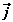form an angle of 45°.## Solution of exercise 9

If {,} is an orthonormal basis, calculate:

1·= 1 · 1 · cos 0° = 1

2·= 1 · 1 · cos 90° = 0

3·= 1 · 1 · cos 90° = 0

4·= 1 · 1 · cos 0° = 1

Did you like the article?(2 votes, average: 5.00 out of 5)Loading...

Emma

I am passionate about travelling and currently live and work in Paris. I like to spend my time reading, gardening, running, learning languages and exploring new places.

Did you like
this resource?

Bravo!